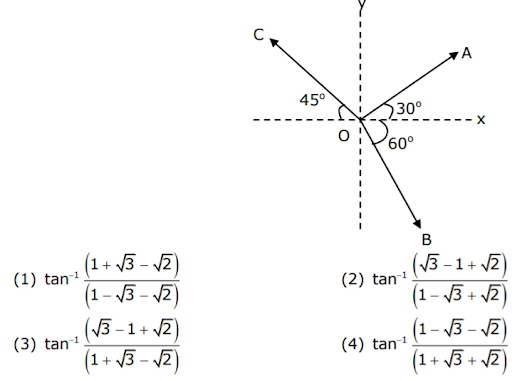# The magnitude of vectors OA, OB and OC in the given figure are equal.

The magnitude of vectors $$\overrightarrow {AB},\overrightarrow {OB}$$ and  $$\overrightarrow{OC}$$ in the given figure are equal. The direction of $$\overrightarrow{OA}+\overrightarrow{OB}-\overrightarrow{OC}$$ with x-axis will be :-